Successfully reported this slideshow.Upcoming SlideShare
×

ofUpcoming SlideShare
The Real Estate Marketing Guide to Keyword Research
Next

Share

# 2014 Online Marketing Guide for Real Estate

Our 2014 Online Marketing Guide for Real Estate details the importance of mobile optimization and content marketing, complete with strategy checklists and key phrases to enhance your 2014 marketing plan.

See all

See all

### 2014 Online Marketing Guide for Real Estate

1. 1. 21 04 ON I EMA K TN G I EF LN R E I G U D OR RA ET T E L SA E MOBL IE MA I NA A EY A MOBL -RE D YMA K T R R OU IE F IN L R EE? 4 6 T A OD Y % 2 0 % I21 N 04 o s ac es s fe rh r ue mo i d v e bl e i s e c e c s ey o o le xl i lfr nn uv i rs ac e e rh o ra e tt s ac e fe l s e e rh s a aep r r do r ef me n o mo i d v e bl e i s e c M be oi l d v e wl ei s i c l ice s gy n rai l n ui e ti l z lc t n& o ai o p r nl es a o peee c rfrn e d tt aa o d le ev r i rl a t e vn e c ne t o tn. T eewl hr i l b moe e r Itrenen t ra y ed mo i bl e dv e ei s c ta hn h m n. u as M be oi l I en t n re t t ﬃ wl r c i a l sras up s d stp ek o I en t n re t tﬃ . r c a teT K A h A E WA Y Mo i - in l mak t gwi h l gv y u bl f e dy rei er n l ep ie o r l b s e steu p r a di 2 1 . ui s h p e h n n 04 n C TN ON E T C A E R Z I C T N MA K TN S ON E T R E IG P R OFY RMA K TN A T OU R E IG SR TG ? TAE Y 7 8 % 6 0 T A OD Y % o C sC if f MO (h e Mak t gO es rei ﬃc r) n b l v c s m c ne t ei e ut o tn e o iteftr o s h uue f mak t g rei n o b s e s s s s me f ui se ue o n fr o c ne t om f o tn mak t g rei n “ o tn mak t gwl eb g rh ne e. C ne t rei ib i e ta v r n l g ” I21 N 04 - a s nD M r F re J yo e es ob s , Moea dmoe r n r c mp ne wl o a is i l i et n si v n d vl i a d ee p g n on ip m ni m l e tg e n c ne tt tg s o tn s ae i r e Mo i -in l bl fe dy er c ne t ib c m o tn wl e o e l i rai lp p l n es g o u r c ny a teT K A h A E WA Y C ne t reigi k yt mo enzn y u o tn mak t n se o d r iig o r srtg a dd iigmo etaf . tae y n r n v r rf c i C E K IT H C LS S U ET E EMA K TN S HS R E IG C E K IT T S A H C LS S O T Y ON T A KI 2 1 R C N 04 ID X B EP G F RE E YL TN N E A L A E O V R I IG S T eb sIXslt n a t-e eae h etD oui s uog n rt o lt gp g so b ot gS O ii a e fr o sn E sn i MO E N B A TF L E IN D R , E U IU D SG D e y u sesl aeta o s o ri tl v h t t ih “ d - - e’0 ”o k Mae v ri ! ma ei t - s lo ? k o e t nh 9 me IT G A E A A Y ISP O R M N E R T D N L TC R G A U iga a a t s rga wl h wy u s n n n l i po rm iso o yc l w a’w riga dw a i ’ h t ok s n n h ts t n Ag o S Opu i wl o sy u sac o d E lgn ib ot o re rh l rn ig &h l y ug to n a k s ep o e fu d n C N E TMA K TN P R O A O TN R E IG E S N S Ce t rpee tt n o teie l rae e rsnai s fh d a o pop cs o ’ t igt e g g rse ty ur r n o n a e e y E IO IL A E D R DT RA C L N A U ea pe dh e t pa o th s sra se to l u te n c ne t o w n t ce t o tn y u a to rae C S O RQ E TO S U T ME U S IN L t u a o teq et n y u i o t l fh u so s o r s l i c s mes s. ol c ne to i ! ut rakV i,o tn tpc o à s K Y R O TMIA IN E WO D P I Z TO Ic d rlv n k y od i tls n l e ee e t e w rsn ie, u t cpin, t d sr t n, t. a t smea eci i sec o po MA EF RMO I D O BL E U emo i -in lfr tn &sot s bl fe dy omat g h r er i p s ta cnb ra o - eg ot h t a e e d nt -o s h VS A C N E T IU L O T N U ev e tushg rslt npo et s i o o r ih eoui rp r d , o y p oo, rsnain, t.oda i la s h tspee tt sect rw n e d o A dsc lh r gb t n t A L f d o i sai ut so L o a n o y u c ne tic dn lt g! o r o tn ( l ig ii s nu sn ) C N IT N B A DN O SS E T R N IG U etesmen me c natno lg , s h a a ,o tc if, o o ecars a o y u c a n l t.cos l f o r h n es l A DN V L E D IG A U B a eo reo tesc l e . h r e rsuc n h o i w b S ae a id s y n we g ,isec n ut k o ld e t , t. r p L T NN I I E IG N S U emo i r gtosoﬁ do t h tok s nt i o lt n u w a fl on s aesy ga o t o r uies r ai b u y u b s s n n E P N IGY U P E E C X A DN O R R S N E E p r n wt PneetIs ga Vn , x ei t i itrs nt rm, ie me h , a ect ice s y u rah t.o n rae o re c Fn a de g g pop cs nsc li s id n n a e rse to o i se, a t n r r rl inhp,n c n et ut e ea o sisa d o v r u t D MANN ME O I A U eao atr i y u se d ma s lclem n o ri ’ o i ts n n me(.. otn o d s o a eg B s C n o. m) o c C N A TIF O TC N O Ce t a C natp g &a as k rae “ o tc” a e l y mae w y u c natnoe s t ﬁ d o r o tc if ay o n C N IT N S A C CT TO S O SS E T E R H I IN A U etesmec natnoo Yh o s h a o tc if n a o ! L cl o ge L cl Bn L cl o a G o l+ o a & ig o a , , LC L LG O A BO Ic d ao asac tr i y u bo ’ n l e lcle rh em n o r lg u s tl (.. otnC n oBo ) ie eg B s t o o d lg LC L O TN O A C NET R v wrs ua t&at cin, triw e i et rns tat si ev e a r o n e lclc lbie, t. o a“ee ris ec t ” R VE E IWS 2 1 W E ST 04 B IE C E K IT H C LS Vsos h udh v a ec l n sac itrso l ae n xel te rh i e e p r n ears a d v e x ei c cos l e i s e l c S CA S LIG O IL E L N 21C TN 0 4 ON E T C E K IT H C LS MO I RE D YML S A C BL F IN L E S ER H S CA S A IGB T O S O IL H RN U T N 2 1 S IL 0 4 OCA C E K IT H C LS O t n: bl-ny i , bl a p pi smo i o lse mo i p , o e t e rso s ed s nse ep ni ei i v g t S OP U I E L GN 21L A 0 4 OC L C E K IT H C LS MO I RE D YWE ST BL F IN L E B IE A k o r l nsow i (ra! e iw o s y u ce tt re ge t rv s n i t ) e G o l,ep ec o ge Y l, t. teT K A h A E WA Y Pa nn o t o r tae y f m we d s nt ln ig u y u srtg ,r o b ei o g lc l E wi ma ey u c ne t r o a S O, l k o r o tn mo e l c h s ea defcie o ei n f t . v e v B I T EK OW EN H N 1E P R T R F 2 1 2 X E T E MS OR 0 4 MA K TN R E IG A T U OMA I TON D I C MP I N RP A A G LF C C EE IS IE Y L MA L A c mmu iainsrtg ta o nc t o t e y ht a s n so t uo tdme s g s e d u a tmae sa e t la so c so r o e as t o e d r u tmes v r e a u t f i . h s me s g s mo n o t me T e e sa e aetpc l " r p d i a r y ial d i e "n y p te ds q e c ta rltst h me e u n e h t eae o tea t n( o la se o k o h ci d wno d b o ) r o c n es nfn e sau ( e r g o v ri u n l tts n ai o n tee do af eta) f h h n f r r lo te e i rcpe t e iin. E i ta aetg ee b s d mal h t r r g rd a e s i of f eeala o c so r f o wh r e d r u tme i i tec n es nfn e. o s n h o v ri u n lF r o e a l, u tmeswh h v x mpe c so r o ae b e wi ac mp n fr n en t h o a y o ma y y aswi g t iee t e r l e df rn l f me s gn ta to ewh h v sa ig h n h s o ae js s n du . ut i e p g C V RI FN E ON E SON U N L C S OME P R ON S UT R ES A T i i me n t s rea a"i a" hs s a t o ev s vs l u rp e e tt no h w ac so r e rs nai f o o u tme i ldtru haco e s l,rm s e ho g ls d ae f o cikn o a Itre l ig n n nen t c a v rs me t osat gaf e d et e n t trn i i r e ta t b yn teofrdp o u t r lo u ig h f e rd c i e o s rie r evc . P ro a aeme n t mo e ra es n s r a t o d le l c so r e g a hc , u tme d mo rp is alwigmak tr t ce t l n o rees o rae c ne t n p o u t ti rdt o tn a d rd cs al e o o a idvd a wi a i gn d n n iiu l t n ma ie h n me hsoy soy a d a , i r, tr, n t b h vo. h s d ti s o l b e a irT e e eal h ud e s c mpeee o g t alw te o lt n u h o l o h mak tr oi gn te ree t ma ie h p ro asn e sa dd s e i a es n ' e d n e i s n r wa ta wi srn te te y h t l t gh n h l e p o u t r evc ofr ga d rd c o s rie f i n en teme s gn u e t s li h sa ig s d o elt . L GT I ON A L K Y OR S EW D S f r s lt n ta alw ot e oui s h t l wa o o mak tr t a tmaerp tie rees o uo t e eiv t ts s s c a e iba t a d a k , u h s mal lss n d i c mp in , t tg es r a ag s wi r g r p h i b s do la b h vo. a e n e d e a ir PC P A p rs o a la t he wod , ha e f te s tre r s te ek y r saee t mey h s e wod r xr l e s e ica d to g u l eyt p cf n ,h u h ni l o i k d ieag e t e l f rfc ae r v ra d a o t f , r ai b t r utdt d a n hg e et s i e e o rwig ih r q ai la s whc alwsfr u ly e d , ih l t o o hg e c n es nta s otr ih r o v ri h n h r o e k y rs e wod . R S ON I ED SG E P SV E I N SM E Ana p o c t we d s n p ra h o b e i g ta a tmaial s is& h t uo t l hf c y t rs e c ne t op o iea e i s o tn t rvd n z o t l iwig&b o ig p i ve n ma rws n e p r n ea rs al e ie x ei c co s ld vc s e (mat h n s tbes ec) s r o e ,a lt, t. p . S ac E gn Mak t g e rh n ie rei n fc s so tep o t no o u e n h rmoi f o we s e tru hice s g b i s ho g n ra i t n ter iblyi s ac e gn h ivs i n e rh n ie i i t rs l p g s e ut a e . s SO E U X S ac E gn Op i z t ni a e rh n ie t ai s mi o s b e o S M ta fc s so u st f E h to u e n ma iuaigwe s e o we np lt n bis r b t p g st ice s ter iblyi a e o n ra e h ivs i n i i t og nc( o - ad s ac e gn r a i n n p i) e rh n ie rs l . e ut s U e E p r n erfr t a s r x ei c ees o e p ro ’ o eal ec p in a d es ns v rlp re t s n o feig fl wiga itrcin e l s ol n n nea t n o o wi ap o u ts rie o t h rd c, evc , r c mp n . o ay C V RI R T ON E SON A E OP I Z TON ( R TMIA I C O) P yP rCiki amo e o a - e- l s c d lf Itre a v rs gwh ri nen t d et i in een a v rs r p yas e ica u t d et es a i p cf mo n i e c t a a i cik do ah i me n d s l e n c ( o l Ad od i aP C Go ge W r s s P p o ie) rvd r. R fr t tep o e so ees o h rc s f e p r nigwi we p g x ei t me n t h b ae d s n c p , f r, n e i , o y of s a d g e v r t n o c ne t oice s ai i s f o tn t n ra e ao tert a whc la sc n et h ae t ih e d o v r . Rsucs eor e ht:s ac e gn w thc m at l/2 5 4 /6 o-e rh r- o - s - o i -xlsv l-oR s ac -td tp/ e rh n ie ac .o /ri e2 6 5 74 -fS ac esN w U eM bl E cu ieyt- e e rhSu y / c e ht:w w s alu ie se .o / a-saes ac -tt/0 0 tp/ w .m l sn ss m c m r l tt-e rhsas7 2 / / b e e ht:w w s at sg t.o / o i - ak t gm bl- ak t ga ayism bl- ak t gsait s tp/ w .m ri ihsc m m bl m rei / o i m rei -n lt / o i m rei -tt i / / n e n e n c e n sc ht:w w d m reig r.o /lgbd3 0 9 / ii l ak t gSrtg --hn s o - e dt- n w fr2 1 tp/ w .c ak t poc m bo /i/4 4 3D gt - rei -tae y3T ig - uN e -oK o -o- 0 4 / n aM n Y ht:w w fre .o /i sjy o d m r/0 31 /8tetp7c ne tm reigte d -h tw l d m n t- 0 4 tp/ w . b sc m st / s n e es2 1 /00 / -o --o tn- ak t -rn sta- i-o iae2 1 / / o e a h n l ht:w w fre .o /i sjy o d m r/0 30 /7tetp7o l em reigte d -h tw l d m n t- 0 4 tp/ w . b sc m st / s n e es2 1 /91 / -o --ni - ak t -rn sta- i-o iae2 1 / / o e a h n n l ht:w w s lsoc m reig lu .o /lg2 1 /85 -o tn- ak t gsas tp/ w .ae fre ak t co dc m bo /0 30 /0 c ne tm rei -tt/ / n n Atbtn ti i s ruo ht:te o n rjc.o /o na piain#c nN 1 14 tp/ h n u poe t m n u /p l t /io - o 7 1 / c c o ht:te o n rjc.o /o nbo s r io - o 0 0 tp/ h n u poe t m n u /rw e/ c nN 2 8 9 / c # ht:te o n rjc.o /o ncik#c nN 1 4 5 tp/ h n u poe t m n u /l /io - o 9 2 / c c ht:te o n rjc.o /o nco d#c nN 3 4 tp/ h n u poe t m n u /lu /io - o 8 1 / c ht:te o n rjc.o /o nd r-o r/io - o 3 4 tp/ h n u poe t m n u /atb ad#c nN 1 4 8 / c ht:te o n rjc.o /o nga h#c nN 1 7 5 tp/ h n u poe t m n u /rp /io - o 8 3 / c ht:te o n rjc.o /o ns ae#c nN 2 3 tp/ h n u poe t m n u /h r/io - o 5 / c ht:te o n rjc.o /o nh n s a e#c nN 1 7 5 tp/ h n u poe t m n u /a d h k /io - o 6 2 / c ht:te o n rjc.o /o nic g i /io - o 5 2 tp/ h n u poe t m n u / o nt #c nN 7 7 / c n o ht:te o n rjc.o /o nln igp g /io - o 6 3 tp/ h n u poe t m n u / dn -a e#c nN 6 0 / c a ht:te o n rjc.o /o nl k#c nN 1 0 6 tp/ h n u poe t m n u /n /io - o 5 6 / c i ht:te o n rjc.o /o nm g p o e#c nN 1 2 0 tp/ h n u poe t m n u / e a h n /io - o 7 5 / c ht:te o n rjc.o /o np du /io - o 2 9 tp/ h n u poe t m n u /o im #c nN 2 6 7 / c ht:te o n rjc.o /o nfn e/io - o 7 2 tp/ h n u poe t m n u / n l c nN 1 3 8 / c u # Cet b a turaett rae euil ls e f e a WE ST S H T B IE T A WO K R B iwi i e a lIXfr etr nn ut t n x beD o b t o le l h d e i mak t g lc le rha dS O F r x et e , rei , asac ,n E . o e p rh l n o p v i l etr o it a s . m s p c ec I OGR H C B P AC S E NF AP I Y L E T R
•#### AlexThorne8

Aug. 9, 2018
•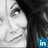#### C0lleenReyn0lds

Oct. 7, 2014
•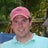#### John_J_Connors

Jul. 12, 2014
•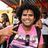#### noddyAnju

Jul. 1, 2014
•#### marchew

May. 17, 2014
•#### paco_espinoza

May. 9, 2014
•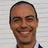#### cesvillas

May. 7, 2014
•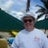#### KevinKnight1

Apr. 22, 2014
•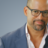#### sethprice

Apr. 1, 2014
•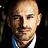#### homesinfo

Mar. 2, 2014
•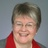#### clkurvers

Mar. 1, 2014
•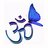#### brokervision

Jan. 19, 2014
•#### DougVanEtten

Jan. 6, 2014
•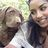Dec. 18, 2013

Total views

3,976

On Slideshare

0

From embeds

0

Number of embeds

34

140

Shares

0